Courses

# GATE Chemistry Mock Test - 2

## 65 Questions MCQ Test GATE Chemistry Mock Test Series | GATE Chemistry Mock Test - 2

Description
This mock test of GATE Chemistry Mock Test - 2 for Chemistry helps you for every Chemistry entrance exam. This contains 65 Multiple Choice Questions for Chemistry GATE Chemistry Mock Test - 2 (mcq) to study with solutions a complete question bank. The solved questions answers in this GATE Chemistry Mock Test - 2 quiz give you a good mix of easy questions and tough questions. Chemistry students definitely take this GATE Chemistry Mock Test - 2 exercise for a better result in the exam. You can find other GATE Chemistry Mock Test - 2 extra questions, long questions & short questions for Chemistry on EduRev as well by searching above.
QUESTION: 1

Solution:
QUESTION: 2

Solution:
QUESTION: 3

### If y = 5x2 + 3, then the tangent at x = 0, y = 3

Solution:
QUESTION: 4

Choose the most appropriate word from the options given below to complete the following sentenceThe principal presented the chief guest with a ______________as token of appreciation.

Solution:
QUESTION: 5

Choose the appropriate word/phrase, out of the four options given below, to complete the following sentence:Frogs______________________.

Solution:
QUESTION: 6

The overwhelming number of people infected with rabies in India has been flagged by the World Health Organization as a source of concern. It is estimated that inoculating 70% of pets and stray dogs against rabies can lead to a significant reduction in the number of people infected with rabies.Which of the following can be logically inferred from the above sentences?

Solution:
QUESTION: 7

A flat is shared by four first year undergraduate students. They agreed to allow the oldest of them to enjoy some extra space in the flat. Manu is two months older than Sravan, who is three months younger than Trideep. Pavan is one month older than Sravan. Who should occupy the extra space in the flat?

Solution:
QUESTION: 8

Find the area bounded by the lines 3x+2y = 14, 2x–3y = 5 in the first quadrant.

Solution:
QUESTION: 9

A straight line is fit to a data set (ln x, y). This line intercepts the abscissa at ln x = 0.1 and has a slope of –0.02. What is the value of y at x = 5 from the fit?

Solution:
QUESTION: 10

S, T, U, V, W, X, Y and Z are seated around a circular table. T is neighbours are Y and V. Z is seated third to the left of T and second to the right of S, U is neighbours are S and Y; and T and W are not seated opposite each other. Who is third to the left of V?

Solution:
QUESTION: 11

Among the following complexes, the ones that show(s) chirality are:
I = [Ru(bipyridyl)3]+, II = [Cr(EDTA)]-, III  = trans[Cr(Cl)2(oxalate)2]3-, IV = cis[Cr(Cl)2(oxalate)2]3-

Solution:
QUESTION: 12

Consider the following statements and choose the correct one(s):
(I) Electron density in the XY plane in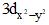orbital is zero            (II) Electron density in the XY plane in 3dz2 orbital is zero
(III) 2s orbital has one nodal surface                                        (IV) For 2pz orbital, YZ is the nodal plane,

Solution:
QUESTION: 13

Choose the correct product of the following reaction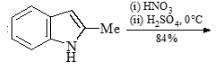Solution:
QUESTION: 14

Which of the following is diastereomer of the given compound?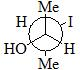Solution:
QUESTION: 15

Which of the following is correct in reference to ferredoxin Fe2S2?
(I) In chloroplasts, Fe2S2 ferredoxins function as electron carriers in the photosynthetic electron transport chain
(II) They are proteins of around one hundred amino acids with four conserved cysteine residues to which the 2Fe-2S cluster is ligated
(III) The iron atoms are tetrahedrally coordinated both by inorganic sulfur atoms and by sulfurs of four conserved cysteine (Cys) residues.

Solution:
QUESTION: 16

Which of the following radial distribution graphs correspond to l = 2 for H atom for the least value of ‘n’ for which l = 2 is allowed?

Solution:
QUESTION: 17

The complex with the most intense color among following is?

Solution:
QUESTION: 18

Select correct statement(s):

Solution:
QUESTION: 19

The rotational partition function of a diatomic molecule with energy levels corresponding to J = 0 and 1 (where ε is a constant).

Solution:
QUESTION: 20

B12H122– has ………… point group:

Solution:
QUESTION: 21

The d-bond is formed via the overlap of (considering z-axis as the inter-nuclear axis):

Solution:
QUESTION: 22

Noble gases (like He, Ne, Ar, Kr etc) are isolated from air. One of the steps is/are

Solution:
QUESTION: 23

Which of the following statement is incorrect?

Solution:
QUESTION: 24

18 electron rule is not followed in one of the following complexes?

Solution:
QUESTION: 25

(II) Actinides, especially those with a small number of 5f-electrons, are prone to hybridization.
(III) Like the lanthanides, all actinides are highly reactive with halogens and chalcogens; however, the actinides react more easily.
(IV) Chemically, actinium is similar to lanthanum, which is explained by their similar ionic radii and electronic structure.

Solution:
QUESTION: 26

The reaction given below is an example of:​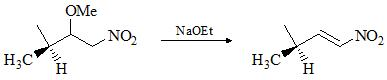Solution:
QUESTION: 27

Choose the correct staetement(s) in reference to Rotational spectroscopy:

Solution:
QUESTION: 28

Choose the major product formed in given chemical reaction sequence: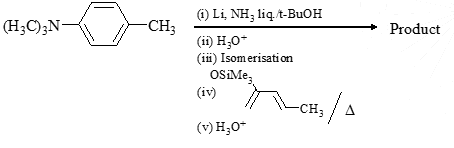Solution:
QUESTION: 29

Major product formed in the following reaction is: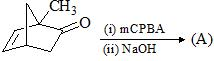Solution:
QUESTION: 30

Choose the correct product of the following reaction: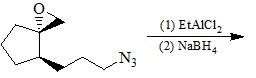Solution:
*Answer can only contain numeric values
QUESTION: 31

The equilibrium constant for the reversible reaction Fe3O4(s) + CO(g) ↔ 3FeO(s) + CO2(g) at 600oC is 1.00. If a mixture initially consisting of 1 mole of Fe3O4, 2 mole of CO, 0.5 mole of FeO and 0.3 mole of CO2 is heated at constant total pressure of 5 atm, how many moles of CO2 will be present at equilibrium?

Solution:
*Answer can only contain numeric values
QUESTION: 32

A closed vessel with rigid walls contain 1 mol of 92U238 and 1 mol of air at 298K. Considering complete decay of 92U238 to 92U206, the ratio of the initial pressure to the final pressure of the system at 298K is____?

Solution:
*Answer can only contain numeric values
QUESTION: 33

Cu metal crystallizes with fcc structure. The density of crystal is 2698 kg/m3 and mass is 26.98 Kg. X-ray of wavelength 1.537 nm are diffracted by the crystal and the Bragg’s angle is 19.20. Identify the crystal plane (m,n,o) which caused this diffraction and give the value (m + n + o):

Solution:
*Answer can only contain numeric values
QUESTION: 34

10 mL of a blood sample (containing Calcium oxalate) is dissolved in an acid. It required 20 mL of 0.001 M KMnO4 (which oxidizes oxalate to CO2). Hence, Ca2+ ion (g) in 10 mL blood is:

Solution:
*Answer can only contain numeric values
QUESTION: 35

The 1H NMR frequency at 1.07 T is 42.4 MHz. If gyromagnetic ratio of 1H and 13C are 27 × 107 and 6.75 × 107 T–1 S–1, respectively, what will be the 13C frequency(MHz) at 1.07 T?

Solution:
QUESTION: 36

Arrange the following in decreasing order of stretching frequency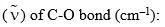(I) Mo(CO)3(NMe3)3                                                     (II) Mo(CO)3[P(OPh)3]3
(III) Mo(CO)3(PMe3)3                                                   (IV) Mo(CO)3(PCl3)3

Solution:
QUESTION: 37

Choose correct statement(s):
(I) Bohr’s radius generally depends upon mass of e, charge on e, n and Z
(II) The probability of finding an e within first Bohr’s radius of 1s orbital of H-atom is less than 30%
(III) Average value of < r2 > in 1s sate of H-atom is 3/2ao.
(IV) Degeneracy of H-atom in n = 3 state including spin is 18.

Solution:
QUESTION: 38

For an enzyme catalyzed reaction, the reaction rate is half of its maximum value when

Solution:
QUESTION: 39

M1 and M2 are the slopes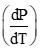of the solid-liquid equilibrium lines in the P-T phase diagrams of Bismuth and CO2 respectively. For P < 10 atm, M1 and M2 are:

Solution:
QUESTION: 40

Assume that for a real system, a real wave function is a linear combination of two orthonormal basis functions where energy integrals are H11 = –15, H22 = –4, H12 = H21 = –1. What would be the ground state and excited wave functions?

Solution:
QUESTION: 41

One mole of an ideal gas is put through a series of changes as shown in the graph in which A, B, C mark the three stages of system. Calculate the pressure at three stages of the system & provide the sum value (PA + PB + PC)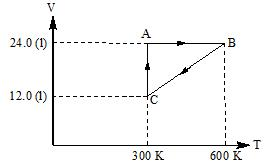Solution:
QUESTION: 42

For a surface inactive substance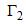(excessive concentration of solute per unit area of surface) is essentially:

Solution:
QUESTION: 43

A metal crystallizes in two cubic phases, fcc and bcc whose unit cell lengths are 3.5Å and 3.0Å resp. What would be the ratio of their densities of fcc to bcc?

Solution:
QUESTION: 44

Which of the following is/are correct in reference to MOT and VB theories?
(I) MO theory underestimates electron correlation by giving equal weightage to ionic and covalent contribution
(II) VB theory overestimates electron correlation by not including ionic contribution
(III) MO theory gives wrong dissociation products
(IV) VB theory is more reliable at larger R than MO theory

Solution:
QUESTION: 45

Inverse spinal structures among following are:   (I) NiFe2O4     (II) ZnFe24   (III) Mn3O4     (IV) CoFe2O4

Solution:
QUESTION: 46

Choose the correct product of the following reaction: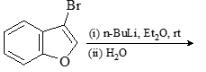Solution:
QUESTION: 47

Choose the correct product of the following reaction: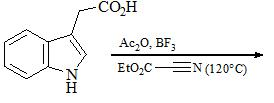Solution:
QUESTION: 48

Select true statement(s) about carbonic anhydrase (a metalloenzyme):

Solution:
QUESTION: 49

Choose the correct product of the following reaction: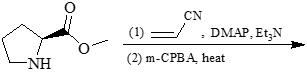Solution:
QUESTION: 50

Match the compounds in Colum-A to their respective point groups in Column -B:

Column-A                                                         Column-B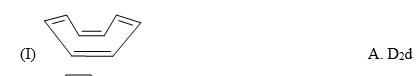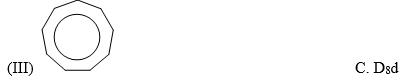Solution:
QUESTION: 51

Consider the following chemical reaction and predict the major product: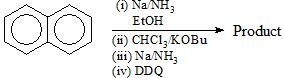Solution:
QUESTION: 52

The reaction in which α-diazoketone cannot be sythesized?

Solution:
QUESTION: 53

Lindane (hexachlorocyclohexane) is an agricultural insecticide that can also be used in the treatment of head lice.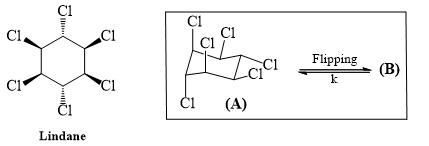Draw the other chair conformation (B) of Lindane and inspect which of the following statements are correct.

Solution:
QUESTION: 54

The major product formed in the following reaction is: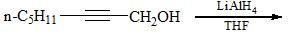Solution:
QUESTION: 55

The X at 1 atm is bubbled through a solution containing a mixture of 1 MY and 1 MZ at 25°C. If the order of reduction potential is Z < Y > X, then:

Solution:
QUESTION: 56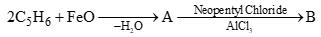The product B is:

Solution:
QUESTION: 57

For one mole of a Vander Waals gas when b = 0 and T = 300 K, the PV vs 1/V plot is shown below. The value of the van der Waals constant a (atm. litre2mol–2) is: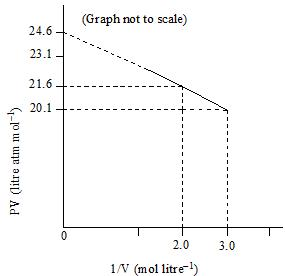Solution:
QUESTION: 58

The radial distribution functions [P(r)] is used to determine the most probable radius, which is used to find the electron in a given orbital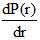for 1s-orbtial of hydrogen like atom having atomic number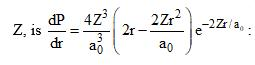Then, which of the following statements is/are correct?

Solution:
*Answer can only contain numeric values
QUESTION: 59

0.5 g of a protein was hydrolyzed into amino acids, one of which is alanine. To this 0.1 g of partially deuterated alanine was H2N-CH-(CD3)-COOH was added. After through mixing, some of the alanine was separated and purified by crystallization. The crystalline alanine contains 0.652 weight percent of D. How many grams of alanine were originally present in 5g of protein?

Solution:
*Answer can only contain numeric values
QUESTION: 60

The mass of metastable ion is produced due to decomposition of F1+ in the following mass fragmentation sequence is: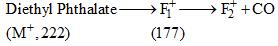Solution:
*Answer can only contain numeric values
QUESTION: 61

The limiting ionic molar conductivities of K+ and Cl- are 73.5 and 76.5 Scm2mol-1, respectively. If the molar conductivity of 0.1 M KCl solution is 130.0 Scm2mol-1, calculate Kohlrausch’s constant (in Scm7/2mol-3/2) for KCl solution:

Solution:
*Answer can only contain numeric values
QUESTION: 62

A sample of 53I131, as iodide ion, was administered to a patient in a carrier consisting of 0.10 mg of stable iodide ion. After 4.00 days, 67.7% of the initial radioactivity was detected in the thyroid gland of the patient. What mass (in mg) of the stable iodide ion had migrated to the thyroid gland?Given T1/2 I131 = 8 days.

Solution:
QUESTION: 63

SZ is represented by the matrix: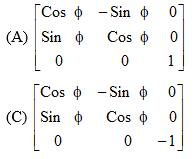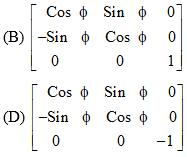Solution:
QUESTION: 64

An ideal gas is allowed to expand both reversibly and irreversibly in an isolated system. If Ti is the initial temperature and Tf is the final temperature, which of the  following statement is correct:

Solution:
QUESTION: 65

Choose correct statement(s)
(I) The energy to first order correction is never below G.S. energy
(II) Second order correction to energy is always negative
(III) Generally non-degenerate perturbation theory is applied to ground state only
(IV) To determine second order energy correction, we have to know only first order correction

Solution: